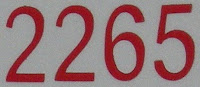## Monday, March 29, 2010

### 2265

2265 = 3 x 5 x 151. It has exactly three distinct palindromic prime factors.

The sum of the digits of 2265 equals the sum of the digits of its prime factors: 2 + 2 + 6 + 5 = 3 + 5 + 1 + 5 + 1 = 15.

The product of the digits of 2265 is eight times the sum of the digits: 2 x 2 x 6 x 5 = 8(2 + 2 + 6 + 5) = 120.

2265 is 14253 in base 6. It uses each positive digit just once.2265 is a year in which January 1 falls on a Sunday.